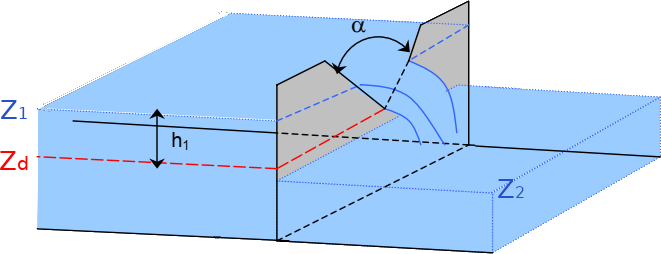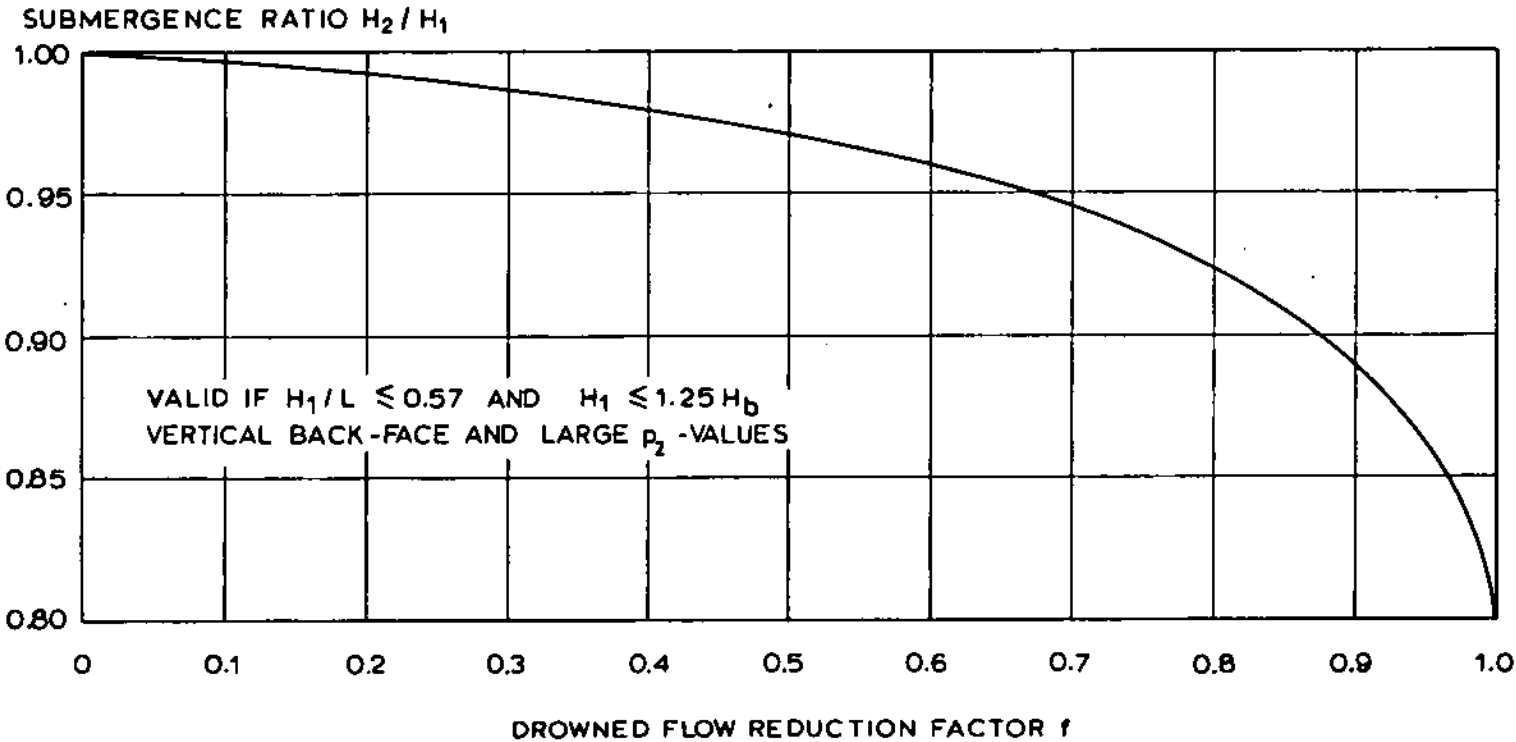# V-notch weir formulaPerspective view of a triangular weir (from CETMEF, 20051)

## Free flow formula

$Q = C_d * \tan \left( \frac{\alpha}{2} \right) \left ( Z_{1} - Z_d \right )^{2.5}$

Avec:

• $$C_d$$ : discharge coefficient
• $$\alpha / 2$$ : half-angle at the apex of the triangle
• $$Z_1$$ : upstream water elevation
• $$Z_d$$ : spill elevation at the tip of the triangle

The discharge coefficient $$C_d$$ depends, among other things, on the thickness of the weir:

• Sharp-crested weir : $$C_d$$ = 1.37
• Broad-crested weir (rounded off $$r > 0.1 * h1$$) : $$C_d$$ = 1.27
• triangular profile weir : (1/2 upstream, 1/2 or 1/5 downstream) : $$C_d$$ = 1.68 and 1.56

## Submergence of a V-notch sharp-crested weir

The weir is submerged as soon as $$Z_{2} > Z_{d}$$ and the Villemonte reduction coefficient is then applied to the discharge calculated in free flow.

## Submergence of a V-notch broad-crested weir

Submergence occurs for $$h_2 / h_1 > 4 / 5$$ with $$h_1 = Z_1 - Z_d$$ and $$h_2 = Z_2 - Z_d$$, and with $$Z_2$$ the downstream water elevation.

The reduction coefficient proposed by Bos (1989) 2 is then applied:Submergence reduction factor for a V-notch broad-crested weir (from Bos, 1989 2)

The abacus is approximated by the following formula:

$K_s = sin(3.9629 (1 - h_2 / h_1)^{0.575})$

1. CETMEF, 2005. Notice sur les déversoirs : synthèse des lois d’écoulement au droit des seuils et déversoirs. Centre d’Études Techniques Maritimes Et Fluviales, Compiègne.

2. Bos, M.G., 1989. Discharge measurement structures., 3rd edition. ed, Publication. International Institute for Land Reclamation and Improvement, Wageningen, The Netherlands.